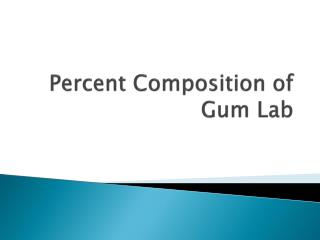DownloadDownload PresentationPercent Composition of Gum Lab

# Percent Composition of Gum Lab

Télécharger la présentation## Percent Composition of Gum Lab

- - - - - - - - - - - - - - - - - - - - - - - - - - - E N D - - - - - - - - - - - - - - - - - - - - - - - - - - -
##### Presentation Transcript

1. Percent Composition of Gum Lab

2. Objective: • Today I will be able to: • Determine the percent composition for each element in a compound • Determine the percent composition of sugar in gum • Evaluation/Assessment: • Informal assessment – monitoring student interactions as they complete the gum lab and the practice • Formal assessment – analyzing student responses to the gum lab, the practice and the exit ticket • Common Core Connection • Make sense of problem and persevere in solving them • Look for and express regularity in repeated reasoning • Attend to precision • Use appropriate tools strategically

3. Lesson Sequence • Evaluate: Warm – Up • Engage and Explore: Percent Composition of Gum Lab • Explain: Percent Composition Notes • Elaborate: Percent Composition Practice • Evaluate: Exit Ticket

4. Warm-Up • An organic compound with a molecular mass of 140 g/mol is 68.54% carbon, 8.63% hydrogen, and 22.83% oxygen. • What is the molecular formula of the compound?

5. Objective • Today I will be able to: • Determine the percent composition for each element in a compound • Determine the percent composition of sugar in gum

6. Homework • Finish Percent Composition Practice • Start studying for quiz on Monday 3/17!!!

7. Agenda • Warm-Up • Percent Composition Notes • Percent Composition of Gum Lab • Percent Composition Practice • Exit Ticket

8. Percent Composition Notes

9. Before we Begin - Lab Set-Up • To calculate the percent composition of sugar in gum • First you need the mass of your gum before you chew it • Take the mass • Chew the gum as we take notes • DO NOT THROW AWAY THE WRAPPER

10. What is Percent Composition? • Percent Composition – is the mass of each element in a compound compared to the entire mass of the compound x 100

11. Percent Composition • Example: • What is the % composition of oxygen and hydrogen in water? • Water = H2O = 18 g/mol 2 g H2 18 g H2O x 100 = 11.1 % Hydrogen 16 g O 18 g H2O 88.9 % Oxygen x 100 =

12. Percent Composition Q: Find the % composition of a compound that contains 2.30 g sodium, 1.60 g oxygen, and .100 g hydrogen in a 4.00 g sample of the compound? 2.30 g Na 4.00 g 1.60 g O 4.00 g x 100 = 40.0 % x 100 = 57.5 % .100 g H 4.00 g x 100 = 2.50 %

13. Percent Composition • A sample of an unknown compound with a mass of .562 g has the following % composition: 13.0% carbon, 2.20% hydrogen, and 84.8% fluorine. When this compound is decomposed into its elements, what mass of each element would be recovered?

14. Percent Composition 13.0% C 100 x .562 g = .0731 g C 2.20 % H 100 .0124 g H x .562 g = x .562 g = .477 g F 84.8 % F 100

15. Percent Composition Lab

16. Lab Directions • Mass your chewed gum. Be sure to put the wrapper down on the balance first • Complete the calculations and the questions • When you finish, you may begin working on the percent composition practice worksheet

17. Percent Composition Practice Complete the practice at your desk. Problems not completed in class will become your homework.

18. Exit Ticket • Write your percent composition of sugar in gum on the board. • We will discuss the results as a class